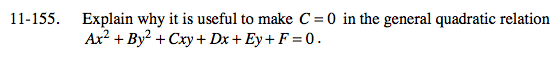### Home > A2C > Chapter Ch11 > Lesson 11.3.3 > Problem11-155

11-155.

Explain why it is useful to make C = 0 in the general quadratic relation Ax2 + By2 + Cxy + Dx + Ey + F = 0. Homework Help ✎Suppose C ≠ 0.
What effect does this have on the process of factoring or manipulating this relation to look like the equation for a conic section?